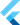# Numbers

on Saturday, 18th of July, 2020

Dart supports sever built in types. These are the most common of the types that represent a single value.

• int
• double
• num
• String
• bool

### int

An `int` is a whole number (integer).

``````int one = 1;
int age = 45;
int year = 2020;

// an int can be negative
int balance = -45;``````

### double

A `double` is a floating-point number, or a number with a decimal point.

``````double percent = .65;
double rating = 4.5;

// a double can be negative
double negativeNumber = -87.42;``````

### num

`num` is a super class of both `int` and `double`. Which means you can use `num` when dealing with numbers that could be an integer or floating-point number. They're best used when you don't know which of the types will be used.

``````num sum;
// now sum has an int value
sum = 4 + 5;

// by adding a double, sum now refers to a double value
sum = sum + 5.5;``````